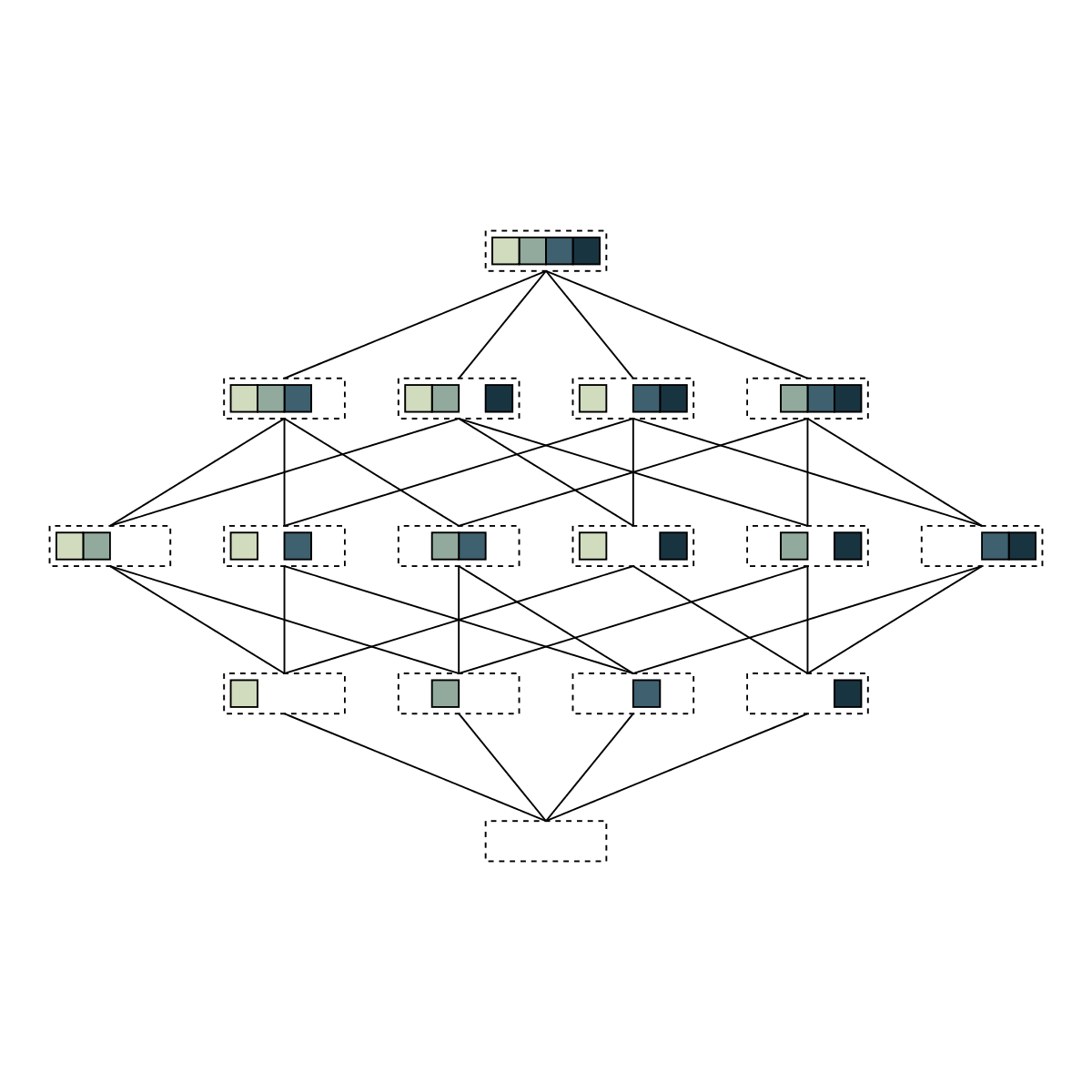Hasse diagram of all subsets of a four-element set.Author: Brent Yorgey
> import Diagrams.Backend.SVG.CmdLine
> {-# LANGUAGE NoMonomorphismRestriction #-}
> import Diagrams.Prelude
> import Data.List
> import Data.Ord (comparing)
> import Data.Function (on)
> import Data.Maybe (fromMaybe)
>
> colors = map sRGB24read["#000000", "#D1DBBD", "#91AA9D", "#3E606F", "#193441", "#000000"]

A subset is represented by the size of the parent set paired with the list of elements in the subset. isSubset tests whether one set is a subset of another; subsetsBySize lists all the subsets of a set of size n, grouped according to size.

> data Subset = Subset Int [Int]
>
> (Subset _ elts1) isSubset (Subset _ elts2) = all (elem elts2) elts1
>
> subsetsBySize :: Int -> [[Subset]]
> subsetsBySize n = map (map (Subset n))
>                 . groupBy ((==) on length)
>                 . sortBy (comparing length)
>                 . subsequences
>                 $[1..n] Draw the elements of a subset, by drawing a colored square for each element present, and leaving a blank space for absent elements. > drawElts n elts = hcat > . map (\i -> if i elem elts > then drawElt i > else strutX 1 > ) >$ [1..n]
>
> drawElt e = unitSquare # fc (colors !! e) # lw thin

Draw a subset by drawing a dashed rectangle around the elements. Note that we also assign a name to the rectangle, corresponding to the elements it contains, which we use to draw connections between subsets later.

> drawSet (Subset n elts) = (    drawElts n elts # centerXY
>                             <> rect (fromIntegral n + 0.5) 1.5
>                                  # dashingG [0.2,0.2] 0
>                                  # lw thin
>                                  # named elts
>                           )

Draw a Hasse diagram by drawing subsets grouped by size in rows, and connecting each set to its subsets in the row below. See the user manual for a more in-depth explanation of how names are used to connect subsets.

> hasseRow = centerX . hcat' (with & sep .~ 2) . map drawSet
>
> hasseDiagram n = setsD # drawConnections # centerXY
>   where setsD = vcat' (with & sep .~ fromIntegral n)
>               . map hasseRow
>               . reverse
>               $subsets > subsets = subsetsBySize n > drawConnections = applyAll connections To generate all the connections, we apply connectSome to each pair of adjacent rows, which calls connect on those pairs where one is a subset of the other. > connections = concat$ zipWith connectSome subsets (tail subsets)
>         connectSome subs1 subs2 = [ connect s1 s2 | s1 <- subs1
>                                                   , s2 <- subs2
>                                                   , s1 isSubset s2 ]

Connect two subsets by looking up the subdiagrams named with their elements, and drawing a line from the upper boundary of one to the lower boundary of the other.

>         connect (Subset _ elts1) (Subset _ elts2) =
>           withNames [elts1, elts2] $\[b1, b2] -> > beneath ((boundaryFrom b1 unitY ~~ boundaryFrom b2 unit_Y) # lw thin) > > example = pad 1.1$ hasseDiagram 4
> main = mainWith (example :: Diagram B)